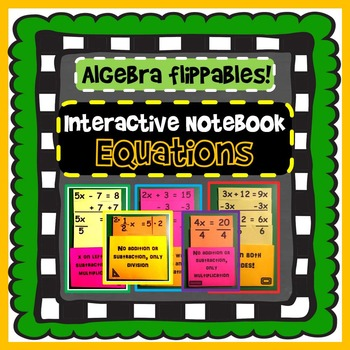# Solving Equations Interactive Notebook Foldables8th - 10th, Homeschool
Subjects
Resource Type
Formats Included
• PDF
Pages
15 pages

### Description

This download includes 8 pocket flippables showing the solving process of 8 different equations (see below). Once put together, each pocket holds a series of different-sized cards that align to show the steps of solving. On the bottoms of each card (out of sight when the cards are all in place) are explanations for each step. Students can add each card to its pocket while reading why each step was taken to solve the equation. The 8 flippables cover equation types with:

x on the left (x + 5 = 9)

x on the left (2x + 5 = 9)

x on the left with subtraction (x - 5 = 9)

x on the left with subtraction and multiplication (2x - 5 = 9)

multiplication only (2x = 10)

x on both sides (2x + 6 = 5x

addition and/or subtraction on both sides (2x + 5 = 4x - 9)

fractions (1/2x = 5)

Related products:

Factoring Flippables!

Equation Spinner BINGO

Equations Mix Match

Interactive Notebook: Graphing in Algebra

Algebra Interactive Notebook BUNDLE

Total Pages
15 pages
Included
Teaching Duration
N/A
Report this Resource to TpT
Reported resources will be reviewed by our team. Report this resource to let us know if this resource violates TpT’s content guidelines.